Technical Article

# Voltage Cells in Parallel

December 14, 2022 by Amna Ahmad

## In this article, learn how to compute the output current and equivalent resistance of voltage cells connected in parallel.

An electrochemical power source is a device that uses an electrochemical reaction to produce electrical energy. An electrochemical power source serves as a backup power source of DC voltage for devices such as electronic intercoms, paging systems, and emergency lighting systems. The most basic type of electrochemical power source is a voltage cell. A voltage cell is a single unit that uses an electrochemical reaction to produce electrical energy. A battery is a group of connected voltage cells that uses an electrochemical reaction to produce electrical energy.

A cell is a unit that produces electricity at a fixed voltage and current level. The current produced by a battery is increased by connecting cells in parallel. When the positive terminal of one voltage cell is connected to the positive of another cell, and the two negative terminals are also connected together, the cells are said to be in parallel.

### Calculating Output Current

Consider two voltage cells connected in parallel, as illustrated in Figure 1(a). If each cell has a terminal voltage of exactly 1.5 V, the output voltage from the parallel combination is also 1.5 V.

Eo = E1 = E2 = E3 = ⋯

If each cell is capable of supplying a maximum current of 1 A, the total output current that can be drawn from the two-cell parallel combination is 2 A. Similarly, for three such cells in parallel, the maximum output current is three times the maximum current per cell. Of course, the actual output current still depends on the output voltage and the load resistance. As shown by Figure 1(b), neglecting cell internal resistances, the output current is,

$I_{o}=\frac{E}{R_{L}}$

But the output current Io is the sum of the individual currents from each cell. Therefore,

Io = I1 + I2 + I3 + ⋯ + In   (1)

For the case of two identical 1.5 V cells in parallel supplying a load resistor of 5Ω (neglecting the internal resistances),

$I_{o}=\frac{E_{C}}{R_{L}}=\frac{1.5V}{5\Omega}=0.3A$

And

I1 = I2 = 0.15A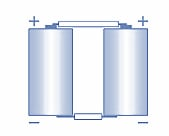##### (a) Two voltage cells connected in parallel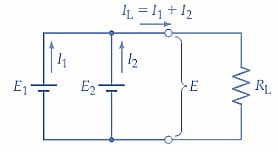##### Figure 1. Parallel-connected cells give an output voltage equal to the terminal voltage of one cell and a maximum output current equal to the sum of the cell maximum current levels.  Image used courtesy of Amna Ahmad

Example.

A 0.2Ω resistance is to be supplied from a parallel-connected battery of 2 V cells. If each cell can supply a maximum current of 0.5 A, determine the number of cells that should be connected in parallel. Assume the cell internal resistances to be negligible.

Solution.

$I_{L}=\frac{E_{C}}{R_{L}}=\frac{2V}{0.2\Omega}=10A$

Number of parallel cells

$N_{P}=\frac{I_{L}}{current\,per\,cell}=\frac{10A}{0.5A}=20$

### Equivalent Resistance of Parallel-connected Cells

In a group of parallel-connected cells, each cell can be represented by its equivalent circuit (E1Rs1, E2Rs2, etc.), as shown in Figure 2(a). The complete battery of cells can be represented by an equivalent circuit with a single source voltage (E) and a single source resistance (Rs) [Figure 2(b)]. E is the terminal voltage of each cell, and Rs equals the parallel combination of the cell internal resistances. For the case of two 1.5 V cells, each having an internal resistance of 0.1 Ω, the equivalent circuit has

E = E1 = E2 = 1.5V

And

$R_{S}=R_{S1}||R_{S2}=\frac{0.1\Omega}{2}=0.05\Omega$

For three identical cells in parallel, the internal resistance of one cell would be divided by three to find the internal resistance of the equivalent circuit,

$R_{S}=R_{S1}||R_{S2}||R_{S3}=\frac{R_{S1}}{3}\,\,\,(2)$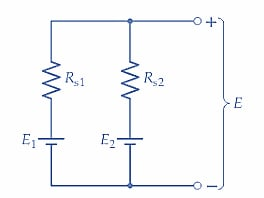##### (a) Equivalent circuit of two parallel-connected cells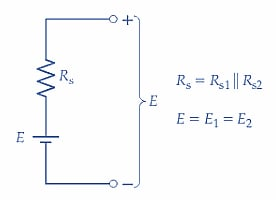##### Figure 2. The equivalent circuit for two parallel-connected cells shows that the internal resistance of the battery is Rs=Rs1||Rs2.  Image used courtesy of Amna Ahmad

Taking the cell internal resistance into consideration, the output current from a group of parallel cells supplying a load resistance RL is

$I_{o}=\frac{E}{R_{S}+R_{L}}\,\,\,(3)$

In some cases, cells connected in parallel may not have voltages that are exactly equal. For example, in the case of the two cells in Figure 1, E1 might be 1.54 V while E2 is 1.49 V. When this occurs, the cell with the largest terminal voltage will tend to discharge through the one with the smaller terminal voltage until both have equal voltages. If secondary (i.e., rechargeable) cells are used, one cell is charged from the other. Cells that do not have closely equal terminal voltages should not be connected in parallel.

### Cell and Battery Capacity

The capacity of a storage battery is stated in ampere-hours (Ah). An ampere-hour is the maximum current a battery can continuously deliver until it is completely discharged for a period of one hour. The capacity of a battery in ampere-hours is found simply by multiplying the number of amperes of current carried by the number of hours the current flows. A battery capable of discharging 2 A of current continuously for a period of 8 hr has a capacity of 2 × 8 = 16 Ah. Likewise, a battery that will deliver a current of 10 A for 12 hr has a capacity of 120 Ah.

Example.

Four cells connected in parallel each have a terminal voltage of E =2 V and internal resistances of Rs=0.1 Ω. Each cell can normally supply a maximum load current of 500 mA for 4 h. Determine the open-circuit output voltage of the parallel combination and the maximum load current that can be supplied. Also, calculate the Ah rating and the internal resistance for the battery of cells.

Solution.

E = E1 = E2 = E3 = E4 = 2V

From equation 1

Io(max) = I1 + I2 + I3 + I4 = 4×500mA = 2A

Ah rating of Battery = (4 cells) × (Ah rating per cell)

= 4 ×(500 mA×4h) = 8 Ah

From equation 2

Rs = Rs1||Rs2||Rs3||Rs4 = 0.1Ω||0.1Ω||0.1Ω||0.1Ω = 0.025Ω

Parallel-connected (similar) cells produce an output voltage equal to the terminal voltage of one cell and can supply a maximum output current equal to the sum of the maximum currents from each cell.

It is very important to ensure that parallel cells are correctly connected. Correct and incorrect ways of connecting cells in parallel are illustrated in Figure 3. All positive terminals should be connected together, and all negative terminals should be connected together, as shown in Figure 3(a).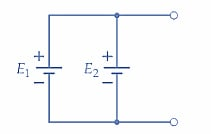##### (a) Circuit diagram of correctly connected parallel cells##### Figure 3. Parallel-connected cells should always be connected positive-to-positive and negative-to-negative. Incorrectly connected cells discharge rapidly. Image used courtesy of Amna Ahmad

Where positive and negative terminals are connected together, as in Figure 3(b), the cells short-circuit each other and discharge rapidly.

### Key Takeaways of Voltage Cells

A simple voltage cell consists of two different metal plates immersed in a liquid electrolyte. Voltage cells can be operated in series, parallel, or series-parallel combinations. The voltage cell or battery’s performance can be defined in terms of the maximum voltage and current it can supply and its ampere-hour (A-h) rating.

Featured image used courtesy of Adobe Stock